# GRE Subject Test: Biochemistry, Cell, and Molecular Biology : Biochemistry

## Example Questions

← Previous 1 3 4 5 6 7 8 9 12 13

### Example Question #1 : Biochemistry

Which of the following statements is not true regarding Gibbs free energy,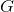If the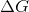of a reaction is positive, the reaction is not favorable and likely requires external energy to proceed

If theof a reaction is less than zero, the reaction is endergonic

If theof a reaction is zero, the reaction is at equilibrium

Theof a reaction is partially dependent on the temperature of the system

Theof a reaction is partially dependent on enthalpy of the system

If theof a reaction is less than zero, the reaction is endergonic

Explanation:

If theof a reaction is less than zero, the reaction will in fact be exergonic. These reactions will be favorable and spontaneous, and energy is released in these reactions. Thus, endergonic is incorrect.

### Example Question #2 : Biochemistry

The equilibrium constant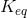of any given chemical reaction is __________ on the temperature and pressure of the reactants and products and is __________ of the reaction rate.

dependent . . . one-half

Independent . . . one-half

independent . . . dependent

dependent . . . dependent

dependent . . . independent

dependent . . . independent

Explanation:

The temperature and pressure of the reactants and products of any given reaction will determine the value of. However, this is entirely dependent of the reaction rate, which is determined by how the concentrations of the reactants and products change over the course of the reaction. Additionally, every reaction is unique, so "one-half" or any other exact metric cannot be applied as the definition.

### Example Question #1 : Biochemistry

Which of the following best describes a reaction that has reached equilibrium?is less than zero, and the forward and reverse reactions are occurring at equal rates.is zero, and the forward and reverse reactions are occurring at equal rates.is zero, and both the forward and reverse reactions have stopped occurring completely.is greater than zero, and the forward and reverse reactions are occurring at equal rates.is zero, and the forward reaction is occurring spontaneously.is zero, and the forward and reverse reactions are occurring at equal rates.

Explanation:

In equilibrium, both the forward and reverse reactions continue to occur, but they do so in a way that is equal and thus, there is no net change of reactants and products in the system.is zero in this case because net changes to the system have ceased, and thus the free energy is no longer in flux.

### Example Question #4 : Biochemistry

__________ reactions have athat is less than zero, and thus they occur spontaneously. This is because the products in these reactions have lower bond energies than the reactants, and when that energy is released it is converted to __________.

exothermic . . . electricity

endothermic . . . heat

exothermic . . . light

exothermic . . . heat

endothermic . . . light

exothermic . . . heat

Explanation:

Exothermic reactions release heat (think exothermic = explosion = creates heat), and this is due to the release of energy from the reactant bonds. These reactions haveless than zero. Endothermic reactions are just the opposite and actually require energy to occur, and therefore do not occur spontaneously nor release heat.

### Example Question #1 : Help With Bonding

Which three elements are capable of participating in hydrogen bonding?

Iron (Fe), oxygen, sodium (Na)

Francium, oxygen, nickel

Fluorine, oxygen, nitrogen

Fluorine, osmium, nitrogen

Francium, osmium, nobelium

Fluorine, oxygen, nitrogen

Explanation:

A hydrogen bond is the weak attraction of a covalently bonded hydrogen to nearby fluorine, oxygen, or nitrogen (FON) atoms. These attractions can occur within a single molecule (intramolecular) or between two distinct molecules in close proximity (intermolecular). Hydrogen bonds give water its cohesiveness and its surface tension.

### Example Question #1 : Biochemistry

The strength of bonds and intermolecular forces, from strong to weak, can be recalled by the use of the mnemonic "I Can't Handle Dirty Vans," which stands for __________.

incomplete, carbon, hardy, direct, van't Hoff

indirect, chorion, high-energy, DeVries, vital

intermediate, central, hybrid, diatomic, variable

isotonic, compound, homologous, dense, valance

ionic, covalent, hydrogen, dipole, van der Waals

ionic, covalent, hydrogen, dipole, van der Waals

Explanation:

Ionic bonds form when one or more electrons from an atom are removed and attached to another atom resulting in positive and negative ions that attract each other. Sodium chloride is an example of an ionic compound. Covalent bonds form when one or more pairs of electrons are shared by two atoms. Hydrochloric acid is an example of a covalent compound. A hydrogen bond is a force of attraction between a hydrogen atom in one molecule and a small atom of high electronegativity (oxygen, nitrogen, or fluorine) in another molecule. Water molecules are capable of intermolecular hydrogen bonds. A dipole-dipole bond is an attractive force between the positive end of one polar molecule and the negative end of another polar molecule. Iodine monochloride molecules show dipole-dipole attraction. Van der Waals forces arise from spontaneous changes in electron density; these occur in all chemical molecules regardless of composition and are extremely weak interactions.

### Example Question #1 : Biochemistry

A mnemonic for remembering whether energy is required or released when chemical bonds are formed is __________.

BARF

LEO says GER

SCAN

RICE

PASS

BARF

Explanation:

Energy is absorbed when bonds break. The energy required to break the bond is absorbed from the surroundings. You have to put energy into a molecule to break its chemical bond.

Energy is released when bonds form. Bond formation represents a stable configuration of atoms. If breaking a bond absorbs energy, forming a bond must release energy; thus, the mnemonic BARF—Break (a bond), Absorb (energy), Release (energy), Form (a bond).

SCAN is the mnemonic for the magnetic field induced in a coil—south clockwise, anticlockwise north. PASS stands for pull, aim, squeeze, sweep—how to use a fire extinguisher. RICE is the treatment for an injury to a joint: rest, ice, compression, elevation. LEO says GER is a mnemonic for loss of electrons is oxidation, gain of electrons is reduction.

### Example Question #1 : Biochemistry

Which of the following forces is strongest between molecules of hydrochloric acid (HCl)?

Hydrogen bonding

Ionic bonding

London dispersion forces

Dipole-dipole interactions

Dipole-dipole interactions

Explanation:

Since hydrochloric acid has a partial positive charge on the hydrogen and a partial negative charge on the chlorine, the molecules will have dipole-dipole interactions between them. Although hydrogen bonding is a stronger force, a hydrogen must be attached to a nitrogen, oxygen, or fluorine in order to exhibit hydrogen bonding.

### Example Question #1 : Biochemistry

Which of the following molecules is not capable of hydrogen bonding?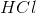Ethanol

Acetic acid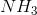Explanation:

Hydrogen bonding is possible when a hydrogen atom is attached to a nitrogen, oxygen, or fluorine. Of the following options, hydrochloric acid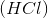is the only option that does not fit the criteria.

### Example Question #1 : Biochemistry

Consider the following reaction: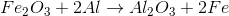Which atom is oxidized in the reaction?

None of the atoms are oxidized

Iron

Oxygen

Aluminum